# Precalculus : Rational Functions

## Example Questions

1 2 3 4 6 Next →

### Example Question #8 : Rational Equations And Partial Fractions

Solve the inequality: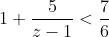Possible Answers:

1 < z < 31

z < 1

No solution

z < 1 or z > 31

Correct answer:

z < 1 or z > 31

Explanation:

First, find the LCD, which is 6(z-1). Then, multiply the entire equation by this: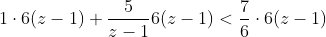This simplifies to: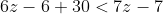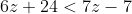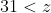Now, make a number line with points at 1 and 31, and vertical lines in between zones: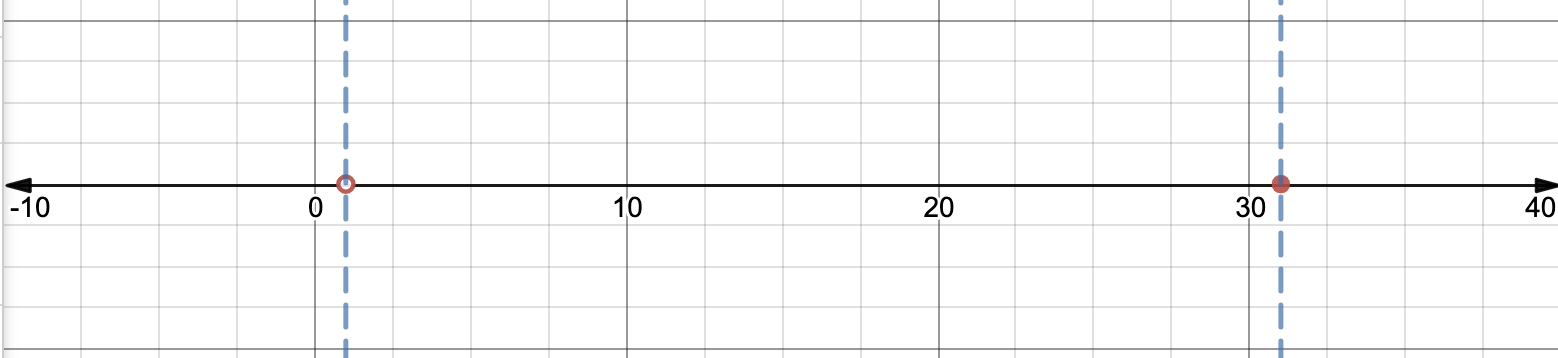Let's test three points, one in each zone:

f(0):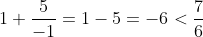f(10):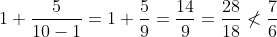f(35):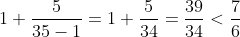Therefore, the inequality is true for values of z such that z < 1 or z > 31.

### Example Question #9 : Rational Equations And Partial Fractions

Decompose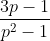into partial fractions.

Possible Answers: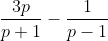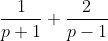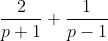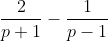Correct answer:Explanation:

Decomposing a fraction into partial fractions shows what fractions were added or subtracted to result in the expression we see. First, factor the denominator.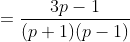Next, we'll express the factored form as the sum of two fractions. We don't know these fractions' numerators, so we'll call them X and Y.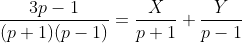Next, eliminate all of the denominators by multiplying both sides of the fraction by the LCD.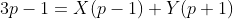Looking back to our original fraction, note that the values p = 1 and b = -1 cannot be plugged in, as they will make the function undefined. However, if we take each of these values and plug them in, we can solve for X and Y.

First, let p=1.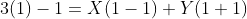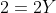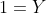Second, let p=-1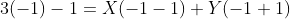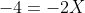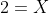Now, substitute these values in for X and Y.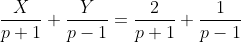Therefore,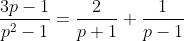.

### Example Question #10 : Rational Equations And Partial Fractions

Decompose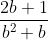into partial fractions.

Possible Answers: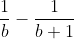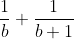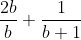Correct answer:Explanation:

Decomposing a fraction into partial fractions shows what fractions were added or subtracted to result in the expression we see. First, factor the denominator.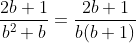Next, we'll express the factored form as the sum of two fractions. We don't know these fractions' numerators, so we'll call them X and Y.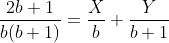Next, eliminate all of the denominators by multiplying both sides of the fraction by the LCD.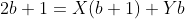Looking back to our original fraction, note that the values b = 0 and b = -1 cannot be plugged in, as they will make the function undefined. However, if we take each of these values and plug them in, we can solve for X and Y.

First, let b=0.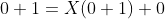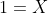Second, let b=-1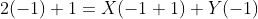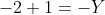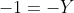Now, substitute these values in for X and Y.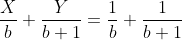Therefore,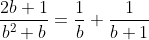.

1 2 3 4 6 Next →

### All Precalculus Resources